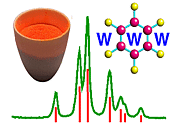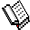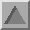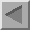A Dimensional SummaryCourse Material IndexSection IndexPrevious Page

A Dimensional Summary

Crystallographers work mainly in 3 dimensions. However, en route to deriving the Structure Factor equation, we passed through cases of lower dimension. The essentials are summarised in the following table; note that if we attribute 0, 1, 2 and 3 dimensions to points, lines, planes, and solids (respectively) we find a dimensional connection between a crystal and its reciprocal lattice (fourth column).

1. Real Space Crystal Defining Parameters 2. Reciprocal Space Dimension (1)+(2)
1D-chain spacing a set of parallel planes 1 + 2 = 3
2D-array spacings a, b angle α set of parallel lines 2 + 1 = 3
3D-crystal spacings a, b, c
angles α, β, γ
3D lattice of points 3 + 0 = 3Course Material IndexSection IndexPrevious Page
 © Copyright 1997-2006.  Birkbeck College, University of London. Author(s): Paul Barnes Martin Vickers